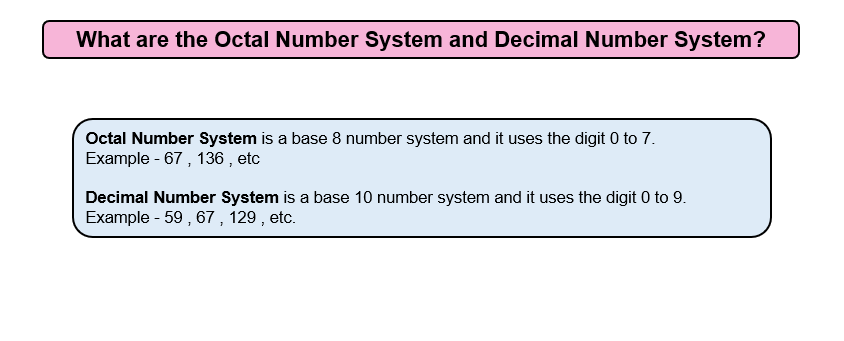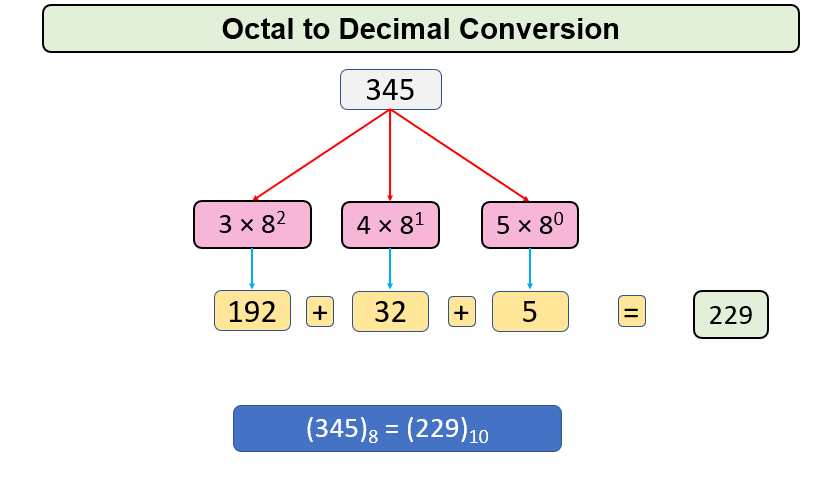# Convert Octal to Decimal

Problem Statement: Given an Octal Number, convert it into a Decimal Number.

Examples:

```Example 1:
Input: 345
Output: 229
Explanation: Decimal equivalent of given Octal expressionis 229

Example 2:
Input: 170
Output: 121
Explanation: Decimal equivalent of given Octal expression is121
```

### Solution

Disclaimer: Don’t jump directly to the solution, try it out yourself first.Approach

In Octal to Decimal Conversion, We will take every digit of the number and multiply it with 8 raised to power i  which will increase by 1 when we move to the next digit and then add it to sum. This task is repeated until n becomes 0.

For Eg, 345

Step 1: Take digit 5, multiply 8 raised to power 0 with it so we get 5 here and now n becomes 34

Step 2: Take digit 4, multiply 8 raised to power 1 with it so we get 32 and add it to the sum which now becomes 37. Also, n becomes 3.

Step 3: Take digit 3 and multiply 8 raised to power 2 with it so we get 192 and add it to sum which now becomes 229. Now n becomes zero.Code:

## C++ Code

``````#include <iostream>
#include <math.h>
using namespace std;
int OctaltoDecimal(int Octal)
{
int Decimal = 0;
int i = 0;
while (Octal != 0)
{
int rem = Octal % 10;
Decimal += rem * pow(8, i);
i++;
Octal /= 10;
}
return Decimal;
}
int main()
{
int Octal = 345;
cout <<"The decimal equivalent of the given octal number is "<<OctaltoDecimal(Octal) << endl;
return 0;
}
``````

Output:

The decimal equivalent of the given octal number is 229

Time Complexity: O(n) where n is the number of digits in the Octal Number.

Space Complexity: O(1)

## Java Code

``````import java.util.*;
public class Main {
public static int OctaltoDecimal(int Octal) {
int Decimal = 0;
int i = 0;
while (Octal != 0) {
int rem = Octal % 10;
Decimal += rem * Math.pow(8, i);
i++;
Octal /= 10;
}
return Decimal;
}
public static void main(String[] args) {
int Octal = 345;
System.out.println("The decimal equivalent of the given octal number is
"+OctaltoDecimal(Octal));
}

}
``````

Output:

The decimal equivalent of the given octal number is 229

Time Complexity: O(n) where n is the number of digits in the Octal Number.

Space Complexity: O(1)

Special thanks to Gurmeet Singh for contributing to this article on takeUforward. If you also wish to share your knowledge with the takeUforward fam, please check out this article